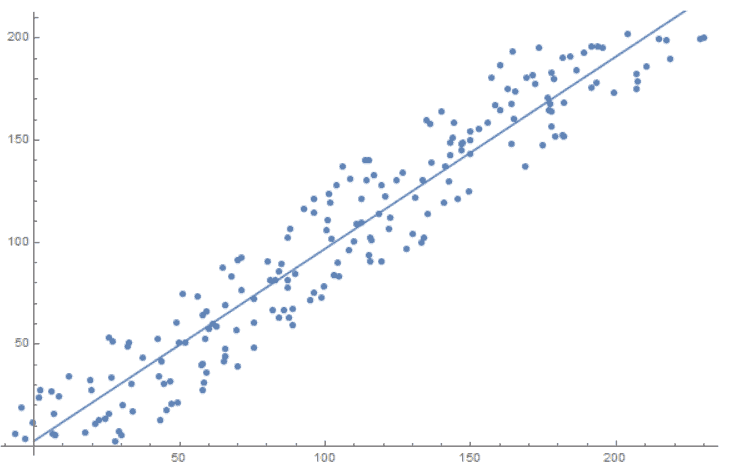## 1. Introduction

In this tutorial, we’ll explain the difference between parameters and hyperparameters in machine learning.

## 2. Parameters

In a broad sense, the goal of machine learning (ML) is to learn patterns from raw data.

ML models are mathematical formalizations of those patterns. For example,is a family of linear models that assumes a linear relationship between the inputand the targetwe approximate with:In it,andare the family’s parameters. Fitting a model, or learning a pattern, means determining the parameters’ values using the data at hand. So, in a specific linear model we use to predictgiven, the parametersandhave specific values, e.g.,and.

The only way to get the parameters is to apply a training algorithm. It returns those values of the parameters that minimize the cost function.

## 3. Hyperparameters

In general, ML follows a simple rule: the better the dataset, the more accurate and precise the models we get. However, a model’s accuracy doesn’t depend only on the data but also on the training algorithm.

The parameters controlling the execution of training algorithms and specifying the model family are called hyperparameters. For example, the learning rate in the gradient descent (GD) algorithm is a hyperparameter. We can set it before seeing the data, and its value affects how GD searches for the parameters. Similarly, the number of hidden layers in a neural network is also a hyperparameter since it specifies the architecture of the network we train.

So, we set hyperparameters in advance. In contrast, we don’t set parameters ourselves. Instead, training algorithms set their values for us.

The search spaces for parameters are usually huge, even infinite. For example,andin the linear models above can take any real value. On the other hand, we usually try only a handful of hyperparameter values in cross-validation. That’s because evaluating a single combination of hyperparameters requires us to complete the model’s training, whereas evaluating parameters requires computing the cost function.

## 4. Summary

Here’s a summary of the differences:

``

## 5. Conclusion

In this article, we explained the difference between the parameters and hyperparameters in machine learning. Whereas parameters specify an ML model, hyperparameters specify the model family or control the training algorithm we use to set the parameters.